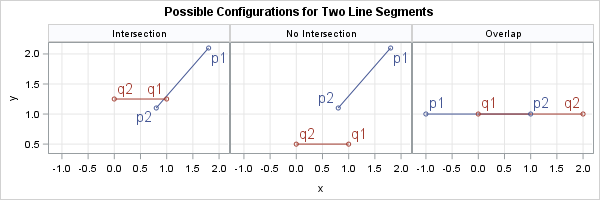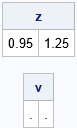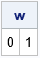Back in high school, you probably learned to find the intersection of two lines in the plane. The intersection requires solving a system of two linear equations. There are three cases: (1) the lines intersect in a unique point, (2) the lines are parallel and do not intersect, or (3) the lines are coincident. Thus, for two lines, the intersection problem has either 1, 0, or infinitely many solutions. Most students quickly learn that the lines always intersect when their slopes are different, whereas the special cases (parallel or coincident) occur when the lines have the same slope.

Recently I had to find the intersection between two line segments in the plane. Line segments have finite extent, so segments with different slopes may or may not intersect. For example, the following panel of graphs shows three pairs of line segments in the plane. In the first panel, the segments intersect. In the second panel, the segments have the same slopes as in the first panel, but these segments do not intersect. In the third panel, the segments intersect in an interval. This article shows how to construct a linear system of equations that distinguishes between the three cases and compute an intersection point, if it exists.### Parameterization of line segments

Let p1 and p2 be the endpoints of one segment and let q1 and q2 be the endpoints of the other. Recall that a parametrization of the first segment is (1-s)*p1 + s*p2, where s ∈ [0,1] and the endpoints are treated as 2-D vectors. Similarly, a parametrization of the second segment is (1-t)*q1 + t*q2, where t ∈ [0,1]. Consequently, the segments intersect if and only if there exists values for (s,t) in the unit square such that
(1-s)*p1 + s*p2 = (1-t)*q1 + t*q2
You can rearrange the terms to rewrite the equation as
(p2-p1)*s + (q1-q2)*t = q1 - p1

This is a vector equation which can be rewritten in terms of matrices and vectors. Define the 2 x 2 matrix A whose first column contains the elements of (p2-p1) and whose second column contains the elements of (q1-q2). Define b = q1 - p1 and x = (s,t). If the solution of the linear system A*x = b is in the unit square, then the segments intersect. If the solution is not in the unit square, the segments do not intersect. If the segments have the same slope, then the matrix A is singular and you need to perform additional tests to determine whether the segments intersect.

### A vector solution for the intersection of segments

As shown above, the intersection of two planar line segments is neatly expressed in terms of a matrix-vector system. In SAS, the SAS/IML language provides a natural syntax for expressing and solving matrix-vector equations. The following SAS/IML function constructs and solves a linear system. For simplicity, this version does not handle the degenerate case of two segments that have the same slope. That case is handled in the next section.

```start IntersectSegsSimple(p1, p2, q1, q2); b = colvec(q1 - p1); A = colvec(p2-p1) || colvec(q1-q2); /* nonsingular when segments have different slopes */ x = solve(A, b); /* x = (s,t) */ if all(0<=x && x<=1) then /* if x is in [0,1] x [0,1] */ return (1-x)*p1 + x*p2; /* return intersection */ else /* otherwise, segments do not intersect */ return ({. .}); /* return missing values */ finish;   /* Test 1: intersection at (0.95, 1.25) */ p1 = {1.8 2.1}; p2 = {0.8 1.1}; q1 = {1 1.25}; q2 = {0 1.25}; z = IntersectSegsSimple(p1,p2,q1,q2); print z;   /* Test 2: no intersection */ p1 = {-1 0.5}; p2 = {1 0.5}; q1 = {0 1}; q2 = {0 2}; v = IntersectSegsSimple(p1, p2, q1, q2); print v;```The function contains only a few statements. The function is called to solve the examples in the first two panels of the previous graph. The SOLVE function solves the linear system (assuming that a solution exists), and the IF-THEN statement tests whether the solution is in the unit square [0,1] x [0,1]. If so, the function returns the point of intersection. If not, the function returns a pair of missing values.

### The general case: Handling overlapping line segments

For many applications, the function in the previous section is sufficient because it handles the generic cases. For completeness the following module also handles segments that have identical slopes. The DET function determines whether the segments have the same slope. If so, the segments could be parallel or collinear. To determine whether collinear segments intersect, you can test for three conditions:

• The endpoint q1 is inside the segment [p1, p2].
• The endpoint q2 is inside the segment [p1, p2].
• The endpoint p1 is inside the segment [q1, q2].

Notice that the condition "p2 is inside [q1,q2]" does not need to be checked separately because it is already handled by the existing checks. If any of the three conditions are true, there are infinitely many solutions (or the segments share an endpoint). If none of the conditions hold, the segments do not intersect. For overlapping segments, the following function returns an endpoint of the intersection interval.

```/* handle all cases: determine intersection of two planar line segments [p1, p2] and [q1, q2] */ start Intersect2DSegs(p1, p2, q1, q2); b = colvec(q1 - p1); A = colvec(p2-p1) || colvec(q1-q2); if det(A)^=0 then do; /* nonsingular system: 0 or 1 intersection */ x = solve(A, b); /* x = (s,t) */ if all(0<=x && x<=1) then /* if x is in [0,1] x [0,1] */ return (1-x)*p1 + x*p2; /* return intersection */ else /* segments do not intersect */ return ({. .}); /* return missing values */ end; /* segments are collinear: 0 or infinitely many intersections */ denom = choose(p2-p1=0, ., p2-p1); /* protect against division by 0 */ s = (q1 - p1) / denom; /* Is q1 in [p1, p2]? */ if any(0<=s && s<=1) then return q1; s = (q2 - p1) / denom; /* Is q2 in [p1, p2]? */ if any(0<=s && s<=1) then return q2; denom = choose(q2-q1=0, ., q2-q1); /* protect against division by 0 */ s = (p1 - q1) / denom; /* Is p1 in [q1, q2]? */ if any(0<=s && s<=1) then return p1; return ({. .}); /* segments are disjoint */ finish;   /* test overlapping segments; return endpoint of one segment */ p1 = {-1 1}; p2 = {1 1}; q1 = {0 1}; q2 = {2 1}; w = Intersect2DSegs(p1, p2, q1, q2); print w;```In summary, by using matrices, vectors, and linear algebra, you can easily solve for the intersection of two line segments or determine that the segments do not intersect. The general case needs some special logic to handle degenerate configurations, but the code that solves the generic cases is straightforward when expressed in a vectorized language such as SAS/IML.

ShareDistinguished Researcher in Computational Statistics

Rick Wicklin, PhD, is a distinguished researcher in computational statistics at SAS and is a principal developer of PROC IML and SAS/IML Studio. His areas of expertise include computational statistics, simulation, statistical graphics, and modern methods in statistical data analysis. Rick is author of the books Statistical Programming with SAS/IML Software and Simulating Data with SAS.

1.Alternative way is expressing these two line by y-y0=k(x-x0) . And solve the linear system as you did. this code didn't handle degenerate configurations.

proc iml;
start IntersectSegsSimple(p1, p2, q1, q2);
k1=(p1-p2)/(p1-p2);
k2=(q1-q2)/(q1-q2);
A = (k1//k2)||{-1,-1};
b = (k1#p1-p1)//(k2#q1-q1);
x = solve(A, b);
return (x);
finish;
/* Test 1: intersection at (0.95, 1.25) */
p1 = {1.8 2.1}; p2 = {0.8 1.1};
q1 = {1 1.25}; q2 = {0 1.25};
z = IntersectSegsSimple(p1,p2,q1,q2);
print z;
quit;

•Yes, exactly. And this coordinate-based approach is the one that you usually see. I think that the vector-based approach is more elegant and easier to construct from the geometry.